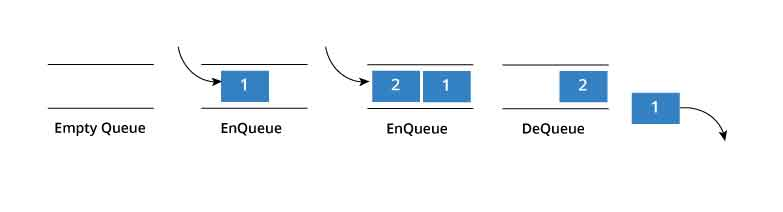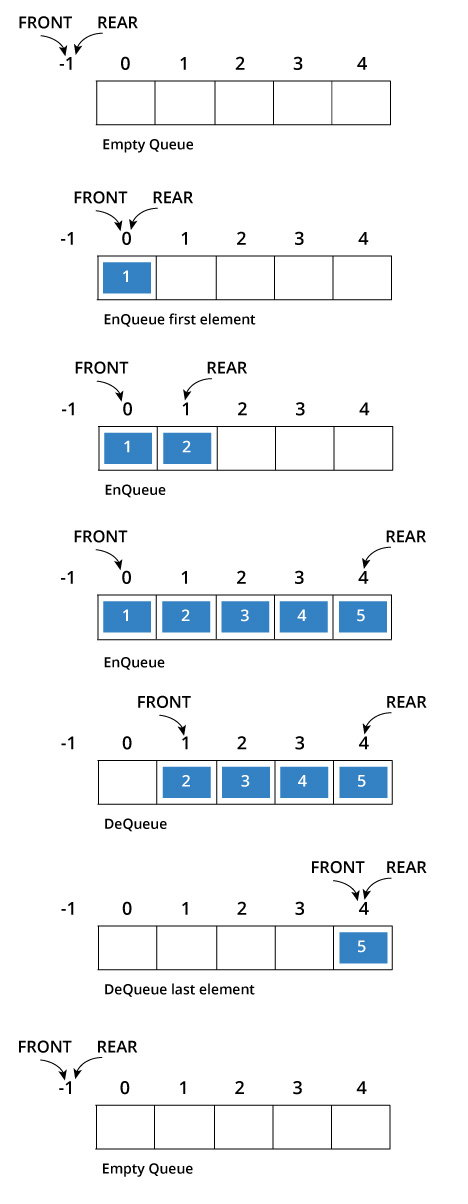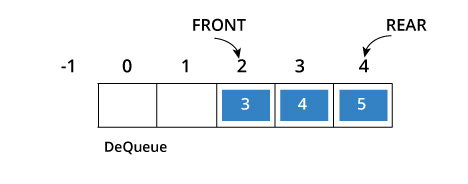# Queue

A queue is a useful data structure in programming. It is similar to the ticket queue outside a cinema hall, where the first person entering the queue is the first person who gets the ticket.

Queue follows the First In First Out(FIFO) rule - the item that goes in first is the item that comes out first too.In the above image, since 1 was kept in the queue before 2, it was the first to be removed from the queue as well. It follows the FIFO rule.

In programming terms, putting an item in the queue is called an "enqueue" and removing an item from the queue is called "dequeue".

We can implement queue in any programming language like C, C++, Java, Python or C#, but the specification is pretty much the same.

## Queue Specifications

A queue is an object or more specifically an abstract data structure(ADT) that allows the following operations:

• Enqueue: Add element to end of queue
• Dequeue: Remove element from front of queue
• IsEmpty: Check if queue is empty
• IsFull: Check if queue is full
• Peek: Get the value of the front of queue without removing it

## How Queue Works

Queue operations work as follows:

1. Two pointers called FRONT and REAR are used to keep track of the first and last elements in the queue.
2. When initializing the queue, we set the value of FRONT and REAR to -1.
3. On enqueing an element, we increase the value of REAR index and place the new element in the position pointed to by REAR.
4. On dequeueing an element, we return the value pointed to by FRONT and increase the FRONT index.
5. Before enqueing, we check if queue is already full.
6. Before dequeuing, we check if queue is already empty.
7. When enqueing the first element, we set the value of FRONT to 0.
8. When dequeing the last element, we reset the values of FRONT and REAR to -1.## Queue Implementation in programming language

The most common queue implementation is using arrays, but it can also be implemented using lists.

### Implementation using C programming

``````#include<stdio.h>
#define SIZE 5

void enQueue(int);
void deQueue();
void display();

int items[SIZE], front = -1, rear = -1;

int main()
{
//deQueue is not possible on empty queue
deQueue();

//enQueue 5 elements
enQueue(1);
enQueue(2);
enQueue(3);
enQueue(4);
enQueue(5);

//6th element can't be added to queue because queue is full
enQueue(6);

display();

//deQueue removes element entered first i.e. 1
deQueue();

//Now we have just 4 elements
display();

return 0;

}

void enQueue(int value){
if(rear == SIZE-1)
printf("\nQueue is Full!!");
else {
if(front == -1)
front = 0;
rear++;
items[rear] = value;
printf("\nInserted -> %d", value);
}
}

void deQueue(){
if(front == -1)
printf("\nQueue is Empty!!");
else{
printf("\nDeleted : %d", items[front]);
front++;
if(front > rear)
front = rear = -1;
}
}

void display(){
if(rear == -1)
printf("\nQueue is Empty!!!");
else{
int i;
printf("\nQueue elements are:\n");
for(i=front; i<=rear; i++)
printf("%d\t",items[i]);
}
}``````

When you run this program, you get the output

```Queue is Empty!!
Inserted -> 1
Inserted -> 2
Inserted -> 3
Inserted -> 4
Inserted -> 5
Queue is Full!!
Queue elements are:
1    2    3    4    5
Deleted : 1
Queue elements are:
2    3    4    5```

### Implementation using C++ programming

``````#include <iostream>
#define SIZE 5

using namespace std;

class Queue {
private:
int items[SIZE], front, rear;

public:
Queue(){
front = -1;
rear = -1;
}

bool isFull(){
if(front == 0 && rear == SIZE - 1){
return true;
}
return false;
}

bool isEmpty(){
if(front == -1) return true;
else return false;
}

void enQueue(int element){
if(isFull()){
cout << "Queue is full";
} else {
if(front == -1) front = 0;
rear++;
items[rear] = element;
cout << endl << "Inserted " << element << endl;
}
}

int deQueue(){
int element;
if(isEmpty()){
cout << "Queue is empty" << endl;
return(-1);
} else {
element = items[front];
if(front >= rear){
front = -1;
rear = -1;
} /* Q has only one element, so we reset the queue after deleting it. */
else {
front++;
}
cout << endl << "Deleted -> " << element << endl;
return(element);
}
}

void display()
{
/* Function to display elements of Queue */
int i;
if(isEmpty()) {
cout << endl << "Empty Queue" << endl;
}
else
{
cout << endl << "Front -> " << front;
cout << endl << "Items -> ";
for(i=front; i<=rear; i++)
cout << items[i] << ""\t";
cout << endl << "Rear -> " << rear << endl;
}
}

};

int main()
{
Queue q;

//deQueue is not possible on empty queue
q.deQueue();

//enQueue 5 elements
q.enQueue(1);
q.enQueue(2);
q.enQueue(3);
q.enQueue(4);
q.enQueue(5);

//6th element can't be added to queue because queue is full
q.enQueue(6);

q.display();

//deQueue removes element entered first i.e. 1
q.deQueue();

//Now we have just 4 elements
q.display();

return 0;
}``````

When you run this program, the output will be

```Queue is empty

Inserted 1

Inserted 2

Inserted 3

Inserted 4

Inserted 5
Queue is full
Front -> 0
Items -> 1    2    3    4    5
Rear -> 4

Deleted -> 1

Front -> 1
Items -> 2    3    4    5
Rear -> 4```

## Limitation of this implementation

As you can see in the image below, after a bit of enqueueing and dequeueing, the size of the queue has been reduced.The indexes 0 and 1 can only be used after the queue is reset when all the elements have been dequeued.

By tweaking the code for queue, we can use the space by implementing a modified queue called circular queue.